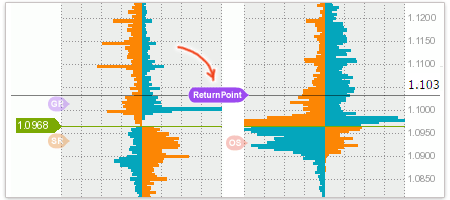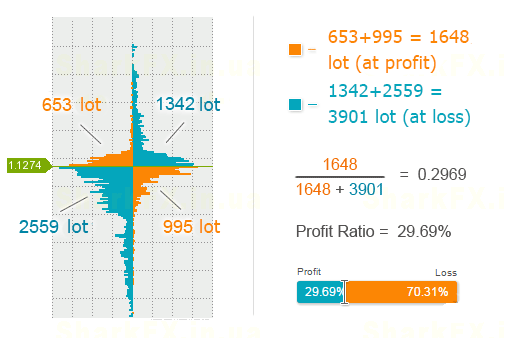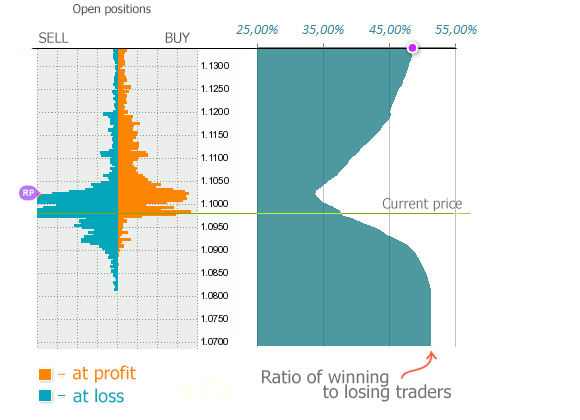# Return Point, or the Point of the Maximum Loss

## Many of you might have noticed a new level appeared in the Order Book tool.Alex Zarevich Forex Sentiment Expert & Analyst

It is called the Return Point:This name suggests that this is a target level, where the price should come back to. However, the name of this level doesn’t fully disclose its essence since it interprets only the strategy but not the origin of the level.

## Return Point

Do you remember what the profit-ratio (the ratio of winning and losing traders) is?

You can find the profit-ratio in the Order Book tool here.

This is how it is calculated:Imagine that each orange or blue pixel represents a trade of 1 lot. If we sum up all the orange pixels, we’ll get the volume of all trades currently in profit. If we divide the resulting figure by the sum of all the pixels (both blue and orange ones), we’ll get the percentage of traders who are in profit now – that is the profit-ratio.

What does this have to do with the Return Point?

The fact is that the Return Point is a derivative of the profit-ratio and points us to the level with a minimum number of winning traders.

Let's explain it with the following example. Imagine that the profit-ratio equals 36% now. In this case, the Return Point will signal where the price should go so that the profit-ratio becomes even smaller.

This is how we identify the Return Point level in the order book:The procedure is generally similar to the one performed earlier with the profit-ratio but only the former is carried out at each level.

On the left, we can see the usual order book showing open positions. The green line stands for the current price, and the black line simulates the order book state in which the current price as if has moved to this level.

Next, we scan the order book and define the percentage of winning traders at each level. The data obtained are presented on the chart to the right.

All we have to do is to identify the point which is the minimum percentage of winning traders. That will be the Return Point.

What’s Interesting in the Return Point for Us?

If we consider the theory of “the market against the crowd”, such a point can serve as a potential target for a future trade or provide a vector (i.e. direction for a trade).

However, there are some nuances that should be taken into account:

• Since the Return Point is derived from the profit-ratio, it will be more efficient in conjunction with the readings of the profit-ratio.
• Most of the time, the Return Point will fluctuate around the price; it will deviate far enough away from the price and generate a signal for a trade only in special cases.
• Since the order book is rather dynamic (new trades are constantly opened and closed), the Return Point will “slightly” float and at times just disappear.

That’s all for now. Should you have any questions, feel free to ask them.

P.S. Any signal, however perfect, has a high probability of being losing.

Keep that in mind.Alex Zarevich Forex Sentiment Expert & Analyst